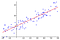# How to Derive an OLS Estimator in 3 Easy Steps

## A Data Scientist’s Must-Know

OLS Estimation was originally derived in 1795 by Gauss. 17 at the time, the genius mathematician was attempting to define the dynamics of planetary orbits and comets alike and in the process, derived much of modern day statistics. Now the methodology I show below is a hell of a lot simpler than the method he used (a redacted Maximum Likelihood Estimation method) but can be shown to be equivalent.

I as a Statistician, owe a lot to the forefathers of Physics.

They derived much of what we know due to necessity. A lot of assumptions had to be made because of their imprecise measuring instruments because unlike today, they couldn’t measure very much or very well at all.

The advances they made in Mathematics and Statistics is almost holy-like given the pedantic depth they explored with such few resources. At the time, very few other people understood their work but it’s because of their advances that we are where we are today.

To the present: OLS Regression is something I actually learned in my second year of undergraduate studies which, as a Mathematical Economist, felt pretty late but I’ve used it ever since.

I like the matrix form of OLS Regression because it has quite a simple closed-form solution (thanks to being a sum of squares problem) and as such, a very intuitive logic in its derivation (that most statisticians should be familiar with).

Moreover, knowing the assumptions and facts behind it has helped in my studies and my career. So from my experience at least, it’s worth knowing really well.

So, from the godfathers of modern Physics and Statistics:

I give to you, OLS Regression.

The goal of OLS Regression is to define the linear relationship between our X and y variables, where we can pose the problem as follows:

Now we can observe y and X, but we cannot observe B. OLS Regression attempts to define Beta.

Beta is very important.

It explains the linear relationship between X and y, which, is easy to visualise directly:The red line is also known as the ‘line of best fit’ where the slope of the red line is what we’re trying to define. [source]

Beta essentially answers the question that “if X goes up, how much can we expect y to go up by?”. Or as in an example, how much does the weight of a person go up by if they grow taller in height?

## 5 OLS Assumptions

Now before we begin the derivation to OLS, it’s important to be mindful of the following assumptions:

1. The model is linear in the parameters
2. No Endogeneity in the model (independent variable X and e are not correlated)
3. Errors are normally distributed with constant variance
4. No autocorrelation in the errors
5. No Multicollinearity between variables

Note: I will not explore these assumptions now, but if you are unfamiliar with them, please look into them or message me as I look to cover them in another article! You can reference this in the meantime.

# Step 1 : Form the problem as a Sum of Squared Residuals

In any form of estimation or model, we attempt to minimise the errors present so that our model has the highest degree of accuracy.

OLS Regression is shown to be MVUE (explained here) but the rationale as to why we minimise the sum of squares (as opposed to say, the sum of cubed) residuals is both simple and complicated (here and here), but boils down to maximising the likelihood of the parameters, given our sample data, which gives an equivalent (albeit requires a more complicated derivation) result.

With this understanding, we can now formulate an expression for the matrix method derivation of the linear regression problem:

which is easy to expand:

# Step 2: Differentiate with respect of Beta.

As we are attempting to minimise the squared errors (which is a convex function), we can differentiate with respect to beta, and equate this to 0. This is quite easy thanks to our objective function being a squared function (and thereby convex), so it’s easy to differentiate:

# Step 3: Rearrange to equal Beta

Now that we have our differentiated function, we can then rearrange it as follows:

and rearrange again to derive our Beta with a nice closed form solution.

And there you have it!

The beauty of OLS regression is that because we’re minimising the sum of squared residuals (to the power 2), the solution is closed form. If it wasn’t to the power 2, we would have to use alternative methods (like optimisers) to solve for Beta. Moreover, changing the power alters how much it weights each datapoint and therefore alters the robustness of a regression problem.

Ultimately, this method of derivation hinges on the problem being a sum of squares problem and the OLS Assumptions, although, these are not limiting reasons not to use this method. Most problems are defined as such and therefore, the above methodology can be (and is) used widely.

However, it’s important to recognise these assumptions exist in case features within the data allude to different underlying distributions or assumptions. For example, if your underlying data has a lot of anomalies, it may be worthwhile using a more robust estimator (like Least Absolute Deviation) than OLS.

Written by